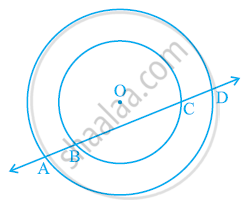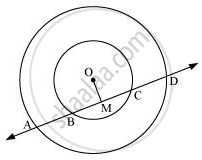# If a line intersects two concentric circles (circles with the same centre) with centre O at A, B, C and D, prove that AB = CD (See given figure) - Mathematics

If a line intersects two concentric circles (circles with the same centre) with centre O at A, B, C and D, prove that AB = CD (See given figure)#### Solution

Let us draw a perpendicular OM on line AD.It can be observed that BC is the chord of the smaller circle and AD is the chord of the bigger circle.

We know that perpendicular drawn from the centre of the circle bisects the chord.

∴ BM = MC ... (1)

And, AM = MD ... (2)

On subtracting equation (2) from (1), we obtain

AM − BM = MD − MC

⇒ AB = CD

Concept: Equal Chords and Their Distances from the Centre
Is there an error in this question or solution?

#### APPEARS IN

NCERT Class 9 Maths
Chapter 10 Circles
Exercise 10.4 | Q 4 | Page 179